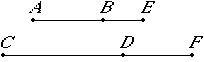# Proposition 103

A straight line commensurable in length with an apotome is an apotome and the same in order.

Let AB be an apotome, B and let CD be commensurable in length with AB.

I say that CD is also an apotome and the same in order with AB.

X.73

Since AB is an apotome, let BE be the annex to it, therefore AE and EB are rational straight lines commensurable in square only.Let it be contrived that the ratio of BE to DF is the same as the ratio of AB to CD. Then one is to one as are all to all. Therefore the whole AE is to the whole CF as AB is to CD.

X.11

But AB is commensurable in length with CD, therefore AE is also commensurable with CF, and BE with DF.

X.13

And AE and EB are rational straight lines commensurable in square only, therefore CF and FD are also rational straight lines commensurable in square only.

V.16

Now since AE is to CF as BE is to DF, therefore, alternately, AE is to EB as CF is to FD. And the square on AE is greater than the square on EB either by the square on a straight line commensurable with AE or by the square on a straight line incommensurable with it.

X.14

If then the square on AE is greater than the square on EB by the square on a straight line commensurable with AE, then the square on CF is also greater than the square on FD by the square on a straight line commensurable with CF.

And, if AE is commensurable in length with the rational straight line set out, then CF is also; if BE, then DF also; and, if neither of the straight lines AE nor EB, then neither of the straight lines CF nor FD.

X.14

But, if the square on AE is greater than the square on EB by the square on a straight line incommensurable with AE, then the square on CF is also greater than the square on FD by the square on a straight line incommensurable with CF.

And, if AE is commensurable in length with the rational straight line set out, then CF is also; if BE, then DF also; and, if neither of the straight lines AE nor EB, then neither of the straight lines CF nor FD. Therefore CD is an apotome and the same in order with AB.

Therefore, a straight line commensurable in length with an apotome is an apotome and the same in order.

Q.E.D.

## Guide

This proposition is not used in the rest of the Elements.